## Bias in Data Science – the Good, the Bad and the Avoidable !?

In recent years, there have been a few prominent examples of accidental bias in machine-learning applications, such as smart phones’ beauty filters (that essentially ended up whitening skin)  or Microsoft’s from-innocent-teen-to-racist-in-24-hours chatbot [2,3]. Examples such as these obviously fell victim to inherently biased data being fed into algorithms too complex to allow for much transparency. Hidden bias continues to be an issue on ubiquitous social media platforms, such as Instagram, whose curators appear to profess themselves both regretful AND baffled . Unfortunately, any model will somewhat regurgitate what it has been fed and interventions at this level of model complexity may prove tricky.

Interestingly, bias itself does not need to be harmful and is often built into a model’s design on purpose, either to address only a subset of the overall population or to model a real-world state, for instance when predicting house prices from its size and number of bedrooms, the model’s bias parameter often represents the average house price in the data set. Thus, we need to distinguish between conscious and unconscious bias in data analysis. Additionally, there is the factor of intent, i.e. is the person conducting the analysis well-intentioned to follow good scientific method or trying to manipulate it to achieve a particular outcome.

In the following, I will only discuss aspects of unintentional and unconscious bias, meaning bias hidden from the researcher or data scientist introducing it. This is by no means an exhaustive discussion, but merely a highlight of some pervasive aspects:

A. Data availability bias

B. Coherent theory bias

C. Method availability/popularity bias

## A. Data availability bias

A. The problem of scientists’ selecting their data out of convenience rather than suitability or representativeness for the current task has been around for a while , e.g. the ideal data set may not be available in machine-readable format or would require higher costs and more time for processing, in short, several obstacles to doing an analysis quickly. For instance, in the area of Natural Language Processing, the major European languages, like English, French and German etc. tend to receive more attention because both data and tools to analyze them are widely available. Similarly, psychology research has mostly focused on so-called WEIRD societies (White, Educated, Industrialized, Rich, Democratic)  and out of convenience often targets the even smaller population of “North American college students” that unsurprisingly have been found to not represent human populations at large.

## B. Coherent theory bias

B. Various studies suggest that we as people strongly favour truths that fit into our pre-existent world view, and why would scientists be exempt from this? Thus, it appears when people analyze data they are often biased by their underlying beliefs about the outcome and are then less likely to yield to unexpected non-significant results . This does not include scientists disregarding new evidence because of conflicting interests . This phenomenon is commonly referred to as confirmation bias or, more fittingly, “myside” bias.

## C. Method availability/popularity bias

C. There is a tendency of hailing new trendy algorithms as one-fits-all solutions for whatever task or application. The solution is presented before examining the problem and its actual requirements. While more complex models are often more powerful, this comes at the cost of interpretability, which in some cases is not advisable. Additionally, some methods, both simple and complex ones, enjoy popularity primarily because they come ready-to-use in one’s favourite programming language.

Going forward…

We as data scientists should:

a. Select our data carefully with our objective in mind. Get to know our data and its limitations.

b. Be honest with ourselves about possible emotional investment in our analyses’ outcomes and resulting conflicts.

c. Examine the problem and its (theoretical) solutions BEFORE making any model design choices.

## References:

 https://www.bbc.com/news/technology-35902104(last accessed 21.10.2020)

 Joseph Rudman (2003) Cherry Picking in Nontraditional Authorship Attribution Studies, CHANCE, 16:2,26-32, DOI: 10.1080/09332480.2003.10554845

 Henrich, Joseph; Heine, Steven J., and Norenzayan, Ara. The Weirdest People in the World? Behavioral and Brain Sciences, 33(2-3):61–83, 2010. doi: 10.1017/S0140525X0999152X.

 Hewitt CE, Mitchell N, Torgerson DJ. Heed the data when results are not significant. BMJ. 2008;336(7634):23-25. doi:10.1136/bmj.39379.359560.AD

 Ioannidis JP. Why most published research findings are false. PLoS Med. 2005;2(8):e124. doi:10.1371/journal.pmed.0020124

## Hiring Data Scientist / Engineer

We are looking for Data Scientist and Engineer.

## Vietnam AI / Data Science LabPlease also visit Vietnam AI Lab

## Motivation

What makes computers useful for us is primarily the ability to solve problems. The procedure in which computers solve a problem is an algorithm.  In the recent context of increasing number of algorithms available for solving data-related problems, there is increasing demand for higher level of understanding of algorithm’s performance in order for data scientists to choose the right algorithms for their problems.

Having a general perception for efficiency of an algorithm would help shaping the thought process for creating or choosing better algorithms. With this intention in mind, I would like to create a series of posts to discuss about what makes a good algorithm in practice, or in short, efficient algorithm. And this article is the first step of the journey.

## Define Efficiency

An algorithm is considered efficient if its resource consumption, also known as computational cost, is at or below some acceptable level. Roughly speaking, ‘acceptable’ means: it will run in a reasonable amount of time or space on an available computer, typically as a function of the size of the input.

There are many ways in which the resources used by an algorithm can be measured: the two most common measures are speed and memory usage. In the next 2 sections, we will be looking at the two different perspectives for measuring efficiency of an algorithm from theoretician and practitioners.

## Theoreticians perspective

Theoreticians are interested in measuring efficiency of algorithm without actually have to run it in several machines and input size. The key idea is that they are not going to actually consider the runtimes of the algorithm on any particular input. Rather, they look at what are known as asymptotic runtimes. Or in other words, they look at how the runtime scale with input size (n) as n gets larger. Does the output scale proportional to n, or proportional to n squared, or maybe exponential in n? In fact, these rate of growth are so different that as long as n is sufficiently large, constant multiples that come from other measures like temporary disk usage, long-term disk usage would be relatively small and neglected.

## Practitioner perspective

While certainly useful, the asymptotic runtime of an algorithm doesn’t tell the whole story. There are algorithms that have good asymptotic runtime, but constants that are so huge that they effectively can’t be used. Ever. Lipton calls them Galactic Algorithms. A galactic algorithm is an algorithm that is wonderful in its asymptotic behavior, but is never used to actual compute anything.

In practice, there are other factors which can affect the efficiency of an algorithm, such as requirements for accuracy and/or reliability. As detailed below, the way in which an algorithm is implemented can also have a significant effect on actual efficiency, though many aspects of this relate to optimization issues.

Implementation issues can also have an effect on efficiency, such as the choice of programming language, or the way in which the algorithm is actually coded, or the choice of a compiler for a particular language, or the compilation options used, or even the operating system being used. In many cases a language implemented by an interpreter may be much slower than a language implemented by a compiler.

## Binomial Theorem

Can you expand on? I guess you would find that is quite easy to do. You can easily find that.

How about the expansion of. It is no longer easy.

It is no longer easy isn’t it. However, if we use Binomial Theorem, this expansion becomes an easy problem.

Binomial Theorem is a very intriguing topic in mathematics and it has wide range of applications.

## Theorem

Letbe real numbers (or complex, or polynomial). For any positive integer, we have:where,Proof:

We will use prove by induction. The base caseis obvious. Now suppose that the theorem is true for the case, that is assume that:we will need to  show that, this is true forLet us consider the left hand side of equation above## We can now apply Pascal’s identity:The equation above can be simplified to:as we desired.

### Example 1:  Power rule in Calculus

In calculus, we always use the power rule thatWe can prove this rule using Binomial Theorem.

Proof:

Recall that derivative for any continuous function f(x) is defined as:Letbe a positive integer and letThe derivative of f(x) is:### Example 2:  Binomial Distribution

Let X be the number of Head a sequence of n independent coin tossing. X is usually model by binomial distribution in probability model. Letbe the probability that a head show up in a toss, and let. The probability that there ishead in the sequence oftoss is:We know that sum of all the probability must equal to 1. In order to show this, we can use Binomial Theorem. We have:Please also check another article Gaussian Samples and N-gram language models ,Bayesian model , Monte Carlo for statistics knowledges.

## Hiring Data Scientist / Engineer

We are looking for Data Scientist and Engineer.

## Vietnam AI / Data Science LabPlease also visit Vietnam AI Lab

## Monte Carlo Simulation

On a nice day 2 years ago, when I was on financial field. My boss sent our team an email. In this email, he would like to us propose some machine learning techniques to predict stock price.

So, after accepting the assignment from my manager, our team begin to research and apply some approaches for prediction. When we talk about Machine Learning, we often think of supervised and unsupervised learning. But one of the algorithms we applied is one that we usually forgotten  however equally highly effective algorithm: Monte Carlo Simulation.

## What is Monte Carlo simulation

Monte Carlo method is a technique that uses random numbers and probability to solve complex problems. The Monte Carlo simulation, or probability simulation, is a technique used to understand the impact of risk and uncertainty in financial sectors, project management, costs, and other forecasting machine learning models.

Now let’s jump into python implementation to see how it applies,

## Python Implementation

In this task we used data of DXG stock dataset from 2017/01/01 to 2018/08/24 and we would like to know what is stock price after 10 days, 1 months and 3 months, respectively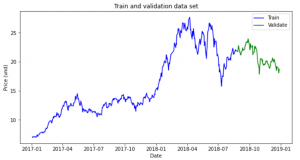We will simulate the return of stock and next price will be calculated by

P(t) = P(0) * (1+return_simulate(t))

Calculate mean and standard deviation of stock returns

miu = np.mean(stock_returns, axis=0)
dev = np.std(stock_returns)

Simulation process

simulation_df = pd.DataFrame()
last_price = init_price
for x in range(mc_rep):
count = 0
daily_vol = dev
price_series = []
price = last_price * (1 + np.random.normal(miu, daily_vol))
price_series.append(price)
for y in range(train_days):
if count == train_days-1:
break
price = price_series[count] * (1 + np.random.normal(miu, daily_vol))
price_series.append(price)
count += 1
simulation_df[x] = price_series

Visualization Monte Carlo Simulation

fig = plt.figure()
fig.suptitle('Monte Carlo Simulation')
plt.plot(simulation_df)
plt.axhline(y = last_price, color = 'r', linestyle = '-')
plt.xlabel('Day')
plt.ylabel('Price')
plt.show()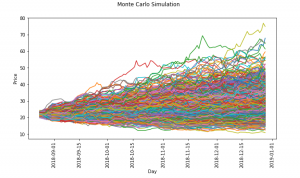Now, let’s check with actual stock price after 10 days, 1 month and 3 months

plt.hist(simulation_df.iloc[9,:],bins=15,label ='histogram')
plt.axvline(x = test_simulate.iloc, color = 'r', linestyle = '-',label ='Price at 10th')
plt.legend()
plt.title('Histogram simulation and last price of 10th day')
plt.show()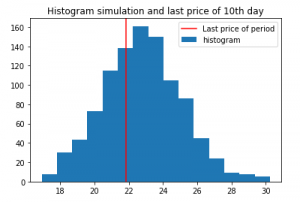We can see the most frequent occurrence price is pretty close with the actual price after 10th

If the forecast period is longer, the results is not good gradually

Simulation for next 1 month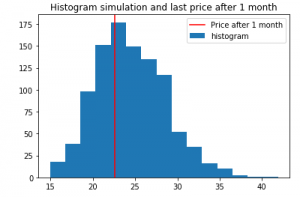After 3 months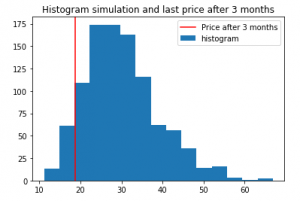## Conclusion

Monte Carlo simulation is used a lot in finance, although it has some weaknesses, but hopefully through this article, you will have a new look on the simulation application for forecasting.

## Reference

 Pratik Shukla, Roberto Iriondo, “Monte Carlo Simulation An In-depth Tutorial with Python”, medium, https://medium.com/towards-artificial-intelligence/monte-carlo-simulation-an-in-depth-tutorial-with-python-bcf6eb7856c8

Please also check Gaussian Samples and N-gram language models,
Bayesian Statistics  for statistics knowledges.

## Hiring Data Scientist / Engineer

We are looking for Data Scientist and Engineer.

## Vietnam AI / Data Science LabPlease also visit Vietnam AI Lab

## Bayesian estimator of the Bernoulli parameter

In this post, I will explain how to calculate a Bayesian estimator. The taken example is very simple: estimate the parameter θ of a Bernoulli distribution.

A random variable X which has the Bernoulli distribution is defined as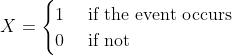with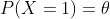In this case, we can write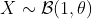.

In reality, the simplest way to eatimate θ is to sample X, count how many time the event occurs, then estimate the probability of occuring of event. This is exactly what the frequestists do.

In this post, I will show how do the Bayesian statisticians estimate θ. Although this doesn’t have a meaningful application, but it helps to understand how do the Bayesian statistics work. Let’s start.

## The posterior distribution of θ

Denote Y as the observation of the event. Given the parameter θ, if we sample the event n time, then the probability that the event occurs k time is (this is called the probability density function of Bernoulli )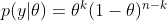In Bayesian statistics, we would like to calculate

By using the Bayesian formula, we haveWith the prior distribution of theta as an Uniform distribution, p(θ) = 1, and it is easy to prove thatwhere Γ is the Gamma distribution. Hence, the posterior distribution isFortunately, this is the density function of the Beta distribution:We use the following properties for evaluating the posterior mean and variance of theta.

If, then## Simulation

In summary, the Bayesian estimator of theta is the Beta distribution with the  mean and variance as above. Here is the Python codes for simulating data and estimating theta

def bayes_estimator_bernoulli(data, a_prior=1, b_prior=1, alpha=0.05):
'''Input:
data is a numpy array with binary value, which has the distribution B(1,theta)    a_prior, b_prior: parameters of prior distribution Beta(a_prior, b_prior)    alpha: significant level of the posterior confidence interval for parameter    Model:
for estimating the parameter theta of a Bernoulli distribution    the prior distribution for theta is Beta(1,1)=Uniform[0,1]    Output:
a,b: two parameters of the posterior distribution Beta(a,b)
pos_mean: posterior estimation for the mean of theta
pos_var: posterior estimation for the var of theta'''
n = len(data)
k = sum(data)
a = k+1
b = n-k+1
pos_mean = 1.*a/(a+b)
pos_var = 1.*(a*b)/((a+b+1)*(a+b)**2)
## Posterior Confidence Interval
theta_inf, theta_sup = beta.interval(1-alpha,a,b)
print('Prior distribution: Beta(%3d, %3d)' %(a_prior,b_prior))
print('Number of trials: %d, number of successes: %d' %(n,k))
print('Posterior distribution: Beta(%3d,%3d)' %(a,b))
print('Posterior mean: %5.4f' %pos_mean)
print('Posterior variance: %5.8f' %pos_var)
print('Posterior std: %5.8f' %(np.sqrt(pos_var)))
print('Posterior Confidence Interval (%2.2f): [%5.4f, %5.4f]' %(1-alpha, theta_inf, theta_sup))
return a, b, pos_mean, pos_var

# Example
n = 129 # sample size
data = np.random.binomial(size=n, n=1, p=0.6)
a, b, pos_mean, pos_var = bayes_estimator_bernoulli(data)

And the result is

Prior distribution: Beta(  1,   1)
Number of trials: 129, number of successes: 76
Posterior distribution: Beta( 77, 54)
Posterior mean: 0.5878
Posterior variance: 0.00183556
Posterior std: 0.04284341
Posterior Confidence Interval (0.95): [0.5027, 0.6703]

In the simulation, we simulated 129 data from the Bernoulli distribution with θ=0.6. And the Bayesian estimation of θ is the posterior mean which is 0.5878.
This is a very simple example of Bayesian estimation. In reality, it is usually tricky to determinate a closed form solution of the posterior distribution from the given prior distribution. In that case, Monte Carlo technique is one of solutions to approximate the posterior distribution.
Please also check Gaussian Samples and N-gram language models for statistics knowledges.

## Hiring Data Scientist / Engineer

We are looking for Data Scientist and Engineer.

## Vietnam AI / Data Science LabPlease also visit Vietnam AI Lab

## Background

In part 1 of my project, I built a unigram language model: it estimates the probability of each word in a text simply based on the fraction of times the word appears in that text.

The text used to train the unigram model is the book “A Game of Thrones” by George R. R. Martin (called train). The texts on which the model is evaluated are “A Clash of Kings” by the same author (called dev1), and “Gone with the Wind” — a book from a completely different author, genre, and time (called dev2).

In this part of the project, I will build higher n-gram models, from bigram (n=2) all the way to 5-gram (n=5). These models are different from the unigram model in part 1, as the context of earlier words is taken into account when estimating the probability of a word.

## Training the model

For a given n-gram model:

The example below shows the how to calculate the probability of a word in a trigram model: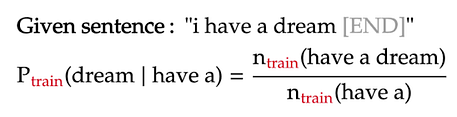For simplicity, all words are lower-cased in the language model, and punctuations are ignored. The presence of the [END] tokens are explained in part 1.

## Dealing with words near the start of sentence

In higher n-gram language models, the words near the start of each sentence will not have a long enough context to apply the formula above. To make the formula consistent for those cases, we will pad these n-grams with sentence-starting symbols [S]. Below are two such examples under the trigram model:

From the above formulas, we see that the n-grams containing the starting symbols are just like any other n-gram. The only difference is that we count them only when they are at the start of a sentence. Lastly, the count of n-grams containing only [S] symbols is naturally the number of sentences in our training text:

## Dealing with unknown n-grams

Similar to the unigram model, the higher n-gram models will encounter n-grams in the evaluation text that never appeared in the training text. This can be solved by adding pseudo-counts to the n-grams in the numerator and/or denominator of the probability formula a.k.a. Laplace smoothing. However, as outlined part 1 of the project, Laplace smoothing is nothing but interpolating the n-gram model with a uniform model, the latter model assigns all n-grams the same probability: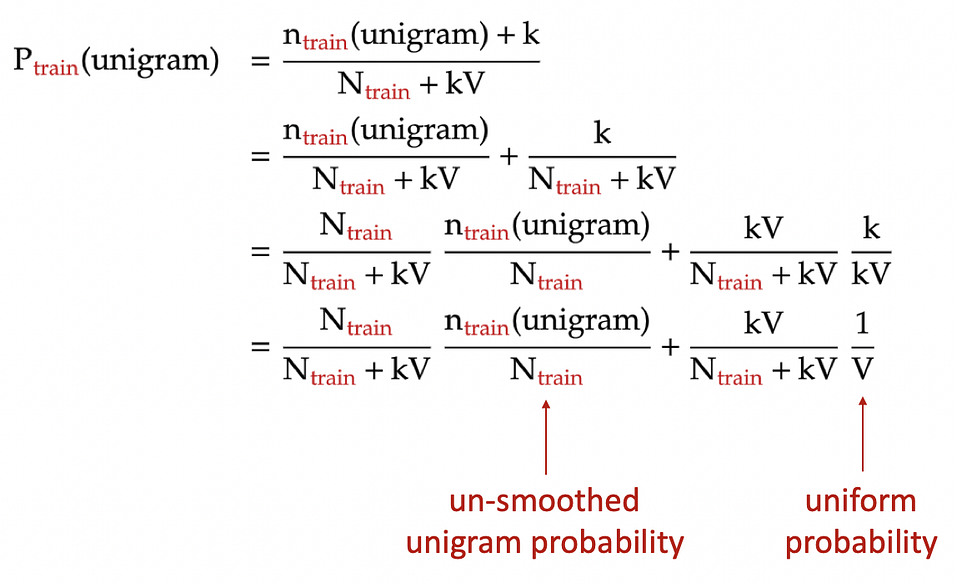Laplace smoothing for unigram model: each unigram is added a pseudo-count of k. N: total number of words in training text. V: number of unique unigrams in training text.

Hence, for simplicity, for an n-gram that appears in the evaluation text but not the training text, we just assign zero probability to that n-gram. Later, we will smooth it with the uniform probability.

## Background

Language modeling — that is, predicting the probability of a word in a sentence — is a fundamental task in natural language processing. It is used in many NLP applications such as autocompletespelling correction, or text generation.

Currently, language models based on neural networks, especially transformers, are the state of the art: they predict very accurately a word in a sentence based on surrounding words. However, in this project, I will revisit the most classic of language model: the n-gram models.

## Data

In this project, my training data set — appropriately called train — is “A Game of Thrones”, the first book in the George R. R. Martin fantasy series that inspired the popular TV show of the same name.

Then, I will use two evaluating texts for our language model:

## What is a unigram?

In natural language processing, an n-gram is a sequence of n words. For example, “statistics” is a unigram (n = 1), “machine learning” is a bigram (n = 2), “natural language processing” is a trigram (n = 3), and so on. For longer n-grams, people just use their lengths to identify them, such as 4-gram, 5-gram, and so on. In this part of the project, we will focus only on language models based on unigrams i.e. single words.

Training the model

A language model estimates the probability of a word in a sentence, typically based on the the words that have come before it. For example, for the sentence “I have a dream”, our goal is to estimate the probability of each word in the sentence based on the previous words in the same sentence: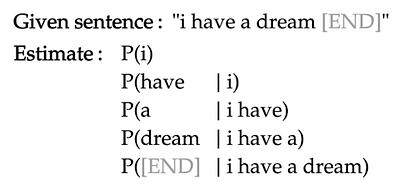For simplicity, all words are lower-cased in the language model, and punctuations are ignored. The [END] token marks the end of the sentence, and will be explained shortly.

The unigram language model makes the following assumptions:

## Background

The goal of this project is to generate Gaussian samples in 2-D from uniform samples, the latter of which can be readily generated using built-in random number generators in most computer languages.

In part 1 of the project, the inverse transform sampling was used to convert each uniform sample into respective x and y coordinates of our Gaussian samples, which are themselves independent standard normal (having mean of 0 and standard deviation of 1):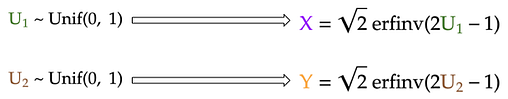Generate x and y coordinates from uniform samples (U₁ and U₂) using inverse transform sampling

However, this method uses the inverse cumulative distribution function (CDF) of the Gaussian distribution, which is not well-defined. Therefore, we approximated this function using the simple Taylor series. However, this only samples accurately near the Gaussian mean, and under-samples more extreme values at both ends of the distribution.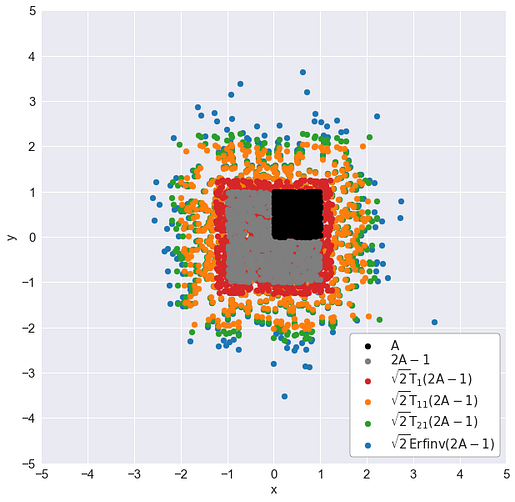A: left-side area (uniformly sampled). Tᵢ: the iᵗʰ-degree Taylor series approximation of the inverse Gaussian CDF: √2 Erfinv(2A-1)

In part 2 of the project, we used the Box-Muller transform, a more direct method to transform the uniform samples into Gaussian ones. The implementation of the algorithm is quite simple, as seen below, but its derivation requires some clever change of variables: instead of sampling Gaussian x and y coordinates for each point, we will sample a uniformly-distributed angle (from 0 to 2π) and an exponentially-distributed random variable that represents half of squared distance of the sample to the origin.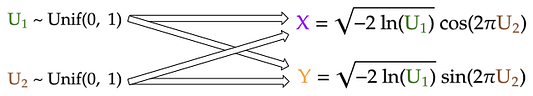Generate x and y coordinates from uniform samples (U₁ and U₂) using Box-Muller transform

In this part of the project, I will present an even simpler method than the above two methods. Even better, this method is one that every statistics students are already familiar with.

## The Central Limit Theorem

It turns out, we can rely on the most fundamental principle of statistics to help us generate Gaussian samples: the central limit theorem. In very simple terms, the central limit theorem states that:

Given n independent random samples from the same distribution, their sum will converge to a Gaussian distribution as n gets large.

Therefore, to generate a Gaussian sample, we can just generate many independent uniform samples and add them together! We then repeat this routine to generate the next standard Gaussian sample until we get enough samples for our x-coordinates. Finally, we just repeat the same steps to generate the y coordinates.

Note that this method will work even if the samples that we start with are not uniform — they are Poisson-distributed, for example. This is because the central limit theorem holds for virtually all probability distributions *cough let’s not talk about the Cauchy distribution cough*.

## Generate Gaussian samples by central limit theorem

To generate, say, 1000 Gaussian sums (n_sums = 1000) where each is the sum of 100 uniform samples (n_additions = 100):

n_additions = 100
n_points = 1000# 0. Initialize random number generator
rng = np.random.RandomState(seed=24) # 1. Generate matrix of uniform samples
uniform_matrix = rng.uniform(size=(n_additions, n_points))# 2. Sum uniform elements down each column to get all Gaussian sums
gaussians = uniform_matrix.sum(axis=0)

We can apply the above method to generate Gaussian samples for each coordinate, using different random number generator for x and for y to ensure that the coordinates are independent from each other. Visualizing the intermediate sums after each addition, we see that:

## Background

In part 1 of this project, I’ve shown how to generate Gaussian samples using the common technique of inversion sampling:

The bad news: the Gaussian inverse CDF is not well-defined, so we have to approximate that function, and the simple Taylor series was used. However, this only samples accurately near the Gaussian mean, and under-samples more extreme values at both ends of the distribution.A: left-side area (uniformly sampled). Tᵢ: the iᵗʰ-degree Taylor series approximation of the inverse Gaussian CDF: √2 Erfinv(2A-1)

Therefore, in this part of the project, we will investigate a more “direct” sampling method that does not depend on the approximation of the Gaussian inverse CDF. This method is called the Box-Muller transform, after the two mathematicians who invented the method in 1958: the British George E. P. Box and the American Mervin E. Muller.Left: George E. P. Box (1919–2013). Right: Mervin E. Muller (1928–2018)

## How does the Box-Muller transform work?

For this project, my goal is to generate Gaussian samples in two dimensions i.e. generating samples whose x and y coordinates are independent standard normals (Gaussian with zero mean and standard deviation of 1). In part 1, I used the inverse Gaussian CDF to generate separately the x and y coordinates from their respective uniform samples (U₁ and U₂):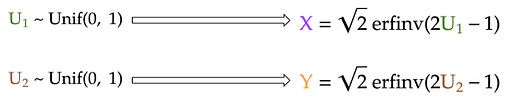Generate x and y coordinates from uniform samples (U₁ and U₂) using inverse transform sampling

For the Box-Muller transform, I will also start with the same two uniform samples. However, I will transform these uniform samples into the x and y coordinates using much simpler formulas: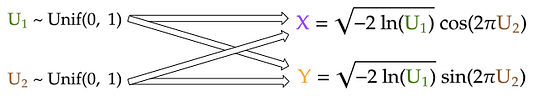Generate x and y coordinates from uniform samples (U₁ and U₂) using Box-Muller transform

Despite the strong coupling between U₁ and U₂ in each of the two formulas above, the generated x and y coordinates, which are both standard Gaussians, are still surprisingly independent from each other! In the derivation of the Box-Muller transform that follows, I will demonstrate why this is indeed this case.

## Derivation of Box-Muller transform

We know that for any two independent random variables x and y, the joint probability density f(x, y) is simply the product of the individual density functions: f(x) and f(y). Furthermore, Pythagorus theorem allows us to combine the x² and y² term in each of the Gaussian density function. This results in the -r²/2 term in the exponential of the joint distribution, where r is the distance from the origin to the 2-D Gaussian sample.

To make it simpler, we then define a variable s that is equal to r²/2. In other words, s is simply the half of squared distance from the origin of our Gaussian sample. Written this way, the joint PDF is simply the product of a constant (1/2π) and an exponential (e⁻ˢ).

## Background

Gaussian sampling — that is, generating samples from a Gaussian distribution — plays an important role in many cutting-edge fields of data science, such as Gaussian processvariational autoencoder, or generative adversarial network. As a result, you often see functions like tf.random.normal in their tutorials.

But, deep down, how does a computer know how to generate Gaussian samples? This series of blog posts will show 3 different ways that we can program our computer (via Python) to do so. You will also see how R and Python generate Gaussian samples using modified versions of some of these methods.

## Starting point: the uniform number generator

Of course, we can’t generate Gaussian samples from thin air. Instead, we start with a random number generator that exists in almost all programming languages: the uniform random number generator. It generates a random number that could take any value between 0 and 1. For Python, the numpy.random module uses the Mersenne twister to generate a uniformly-distributed float that is in the interval [0.0, 1.0).

Since Gaussians are better visualized in 2 dimensions — we are all familiar with the Gaussian “blob” in the xy-plane — I will demonstrate the 3 sampling methods in 2-D, especially since one of the methods do in fact generate Gaussians in two dimensions at the same time.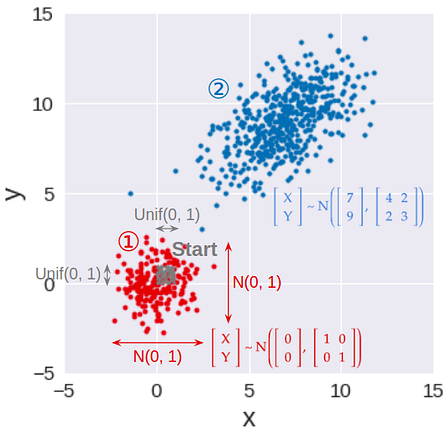Step 1: Generate standard Gaussian samples in 2-D. Step 2: Transform standard Gaussian samples to have given means, variances, and covariance between x and y

As a result, this series is broken down into 3 parts (see accompanying image):

## Method 1: Inverse transform sampling

This is the most basic, yet most common, way to convert a uniform random sample into a random sample of any distribution, including Gaussian. This method works by applying the inverse function of the Gaussian CDF (cumulative distribution function) to transform a uniform sample to a Gaussian sample.

To make sure that the Gaussian samples for the x- and y-coordinates are independent, we can use two different uniform samples, one for x (U₁), and one for y (U₂). These two uniform samples can be generated using two different random number generators (two different RandomState initialized by different seeds, for example) so that they are independent in the first place.